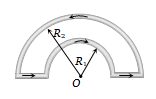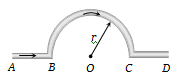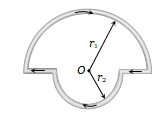A current i ampere flows in a circular arc of wire whose radius is R, which subtend an angle $3\mathrm{\pi }}{2}$ radian at its centre. The magnetic induction B at the centre is :

(a) $\frac{{\mu }_{0}i}{R}$

(b) $\frac{{\mathrm{\mu }}_{0}\mathrm{i}}{2\mathrm{R}}$

(c)$\frac{2{\mathrm{\mu }}_{0}\mathrm{i}}{\mathrm{R}}$

(d) $\frac{3{\mathrm{\mu }}_{0}\mathrm{i}}{8{\mathrm{R}}_{}}$

Concept Questions :-

Magnetic field due to various cases
High Yielding Test Series + Question Bank - NEET 2020

Difficulty Level:

A straight section PQ of a circuit lies along the X-axis from x=$\frac{-\mathrm{a}}{2}$ to x= $\frac{\mathrm{a}}{2}$ and carries a steady current i. The magnetic field due to the section PQ at a point X = + a will be:

1. Proportional to a             2. Proportional to ${a}^{2}$
3. Proportional to $\frac{1}{a}$           4. Zero

Concept Questions :-

Magnetic field due to various cases
High Yielding Test Series + Question Bank - NEET 2020

Difficulty Level:

The magnetic induction at a point P which is distant 4 cm from a long current carrying wire is ${10}^{-8}$ Tesla . The field of induction at a distance 12 cm from the same current would be :
(a)   $3.33×{10}^{-9}$ Tesla

(b) 1.11$×{10}^{-4}$ Tesla
(c)    $3×{10}^{-3}$     Tesla

(d)  $9×{10}^{-2}$    Tesla

Concept Questions :-

Magnetic field due to various cases
High Yielding Test Series + Question Bank - NEET 2020

Difficulty Level:

Two straight horizontal parallel wires are carrying the same current in the same direction, d is the distance between the wires. You are provided with a small freely suspended magnetic needle. At which of the following positions will the orientation of the needle be independent of the magnitude of the current in the wires:

(a) At a distance d/2 from any of the wires in any plane

(b) At a distance d/2 from any of the wires in the horizontal plane

(c) Anywhere on the circumference of a vertical circle of radius d and centre halfway between the wires

(d) At points halfway between the wires in the horizontal plane

High Yielding Test Series + Question Bank - NEET 2020

Difficulty Level:

A circular coil of radius R carries an electric current. The magnetic field due to the coil at a point on the axis of the coil located at a distance r from the centre of the coil, such that r >> R, varies as

(a) $\frac{1}{r}$                                           (b) $\frac{1}{{r}^{3/2}}$
(c) $\frac{1}{{r}^{2}}$                                           (d) $\frac{1}{{r}^{3}}$

Concept Questions :-

Magnetic field due to various cases
High Yielding Test Series + Question Bank - NEET 2020

Difficulty Level:

The magnetic induction due to an infinitely long straight wire carrying a current i at a distance r from the wire is given by

(a) $\left|B\right|=\left(\frac{{\mu }_{0}}{4\mathrm{\pi }}\right)\frac{2i}{r}$                                                 (b) $\left|B\right|=\left(\frac{{\mu }_{0}}{4\mathrm{\pi }}\right)\frac{r}{2i}$
(c)  $\left|B\right|=\left(\frac{4\mathrm{\pi }}{{\mu }_{0}}\right)\frac{2i}{r}$                                                 (d) $\left|B\right|=\left(\frac{4\mathrm{\pi }}{{\mu }_{0}}\right)\frac{r}{2i}$

Concept Questions :-

Magnetic field due to various cases
High Yielding Test Series + Question Bank - NEET 2020

Difficulty Level:

The magnetic induction at the centre O in the figure shown is:1. $\frac{{\mathrm{\mu }}_{0}\mathrm{i}}{4}\left(\frac{1}{{\mathrm{R}}_{1}}-\frac{1}{{\mathrm{R}}_{2}}\right)$                                   2. $\frac{{\mathrm{\mu }}_{0}\mathrm{i}}{4}\left(\frac{1}{{\mathrm{R}}_{1}}+\frac{1}{{\mathrm{R}}_{2}}\right)$

3.                                       4. $\frac{{\mathrm{\mu }}_{0}\mathrm{i}}{4}\left({\mathrm{R}}_{1}+{\mathrm{R}}_{2}\right)$

Concept Questions :-

Magnetic field due to various cases
High Yielding Test Series + Question Bank - NEET 2020

Difficulty Level:

In the figure, shown the magnetic induction at the centre of the arc due to the current in portion AB will be(a) $\frac{{\mathrm{\mu }}_{0}\mathrm{i}}{\mathrm{r}}$                       (c)  $\frac{{\mathrm{\mu }}_{0}\mathrm{i}}{4\mathrm{r}}$

(b) $\frac{{\mathrm{\mu }}_{0}\mathrm{i}}{2\mathrm{r}}$                        (d) Zero

Concept Questions :-

Magnetic field due to various cases
High Yielding Test Series + Question Bank - NEET 2020

Difficulty Level:

Two concentric circular coils of ten turns each are situated in the same plane. Their radii are 20 and 40 cm and they carry respectively 0.2 and 0.3 ampere current in opposite direction. The magnetic field in $weber/{m}^{2}$ at the centre is :

(a) $\frac{35}{4}{\mu }_{0}$                              (b) $\frac{{\mu }_{0}}{80}$
(c)  $\frac{7}{80}{\mu }_{0}$                              (d) $\frac{5}{4}{\mu }_{0}$

Concept Questions :-

Magnetic field due to various cases
High Yielding Test Series + Question Bank - NEET 2020

Difficulty Level:

In the figure shown there are two semicircles of radii ${\mathrm{r}}_{1}$ and ${\mathrm{r}}_{2}$ in which a current i is flowing. The magnetic induction at the centre O will be :(a)  $\frac{{\mathrm{\mu }}_{0}\mathrm{i}}{\mathrm{r}}\left({\mathrm{r}}_{1}+{\mathrm{r}}_{2}\right)$

(b)  $\frac{{\mathrm{\mu }}_{0}\mathrm{i}}{4}\left[\frac{{\mathrm{r}}_{1}+{\mathrm{r}}_{2}}{{\mathrm{r}}_{1}{\mathrm{r}}_{2}}\right]$

(c)  $\frac{{\mathrm{\mu }}_{0}\mathrm{i}}{4}\left({\mathrm{r}}_{1}-{\mathrm{r}}_{2}\right)$

(d) $\frac{{\mathrm{\mu }}_{0}\mathrm{i}}{4}\left[\frac{{\mathrm{r}}_{2}-{\mathrm{r}}_{1}}{{\mathrm{r}}_{1}{\mathrm{r}}_{2}}\right]$

Concept Questions :-

Magnetic field due to various cases